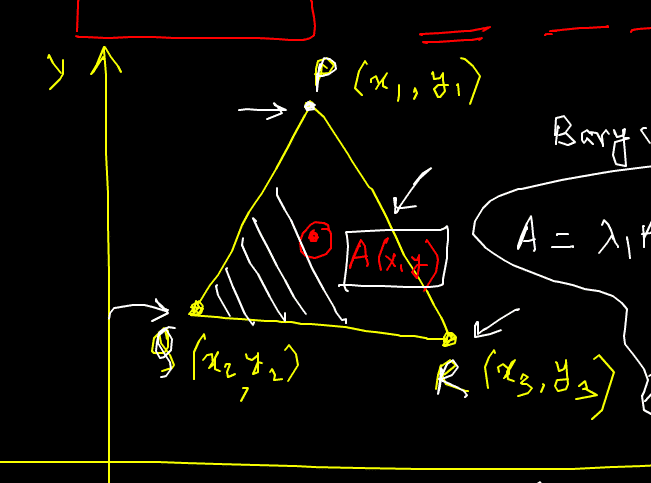How Cheenta works to ensure student success?
Explore the Back-Story

# Barycentric Coordinates - I.S.I 2014 Problem 2The Setup:

Points inside a triangle are 'related' algebraically to points at the vertices. We introduce the idea using a problem from I.S.I. B.Stat 2014.

Problem: Let $PQR$ be a triangle. Take a point $A$ on or inside the triangle. Let $f(x, y) = ax + by + c$. Show that f(A)≤max f(P),f(Q),f(R)

Also Visit: I.S.I & C.M.I Entrance Program

Discussion:

Part 1

# Part 2

The Setup:

Points inside a triangle are 'related' algebraically to points at the vertices. We introduce the idea using a problem from I.S.I. B.Stat 2014.

Problem: Let $PQR$ be a triangle. Take a point $A$ on or inside the triangle. Let $f(x, y) = ax + by + c$. Show that f(A)≤max f(P),f(Q),f(R)

Also Visit: I.S.I & C.M.I Entrance Program

Discussion:

Part 1

# Part 2

This site uses Akismet to reduce spam. Learn how your comment data is processed.

### 3 comments on “Barycentric Coordinates - I.S.I 2014 Problem 2”

1.Parag dey says:

Way! Easy sir Who is ani2000 in art of solving problems forum!!!!

2.Parag dey says:

Way! Easy sir Who is ani2000 in art of solving problems forum!!!! Apmo(asia pacific mathematics Olympiad) is on 13th.

1.Ashani Dasgupta says:

I do not know who that is. Are you participating in APMO? All the best for that

### Knowledge Partner